# 终于有人把排序算法讲明白了• 冒泡排序（bubble sort）
• 归并排序（merge sort）
• 插入排序（insertion sort）
• 希尔排序（shell sort）
• 选择排序（selection sort）

## 01 在Python中交换变量

``````var1 = 1
var2 = 2
var1,var2 = var2,var1

print(var1,var2)``````

``2 1``

## 02 冒泡排序

• 理解冒泡排序背后的逻辑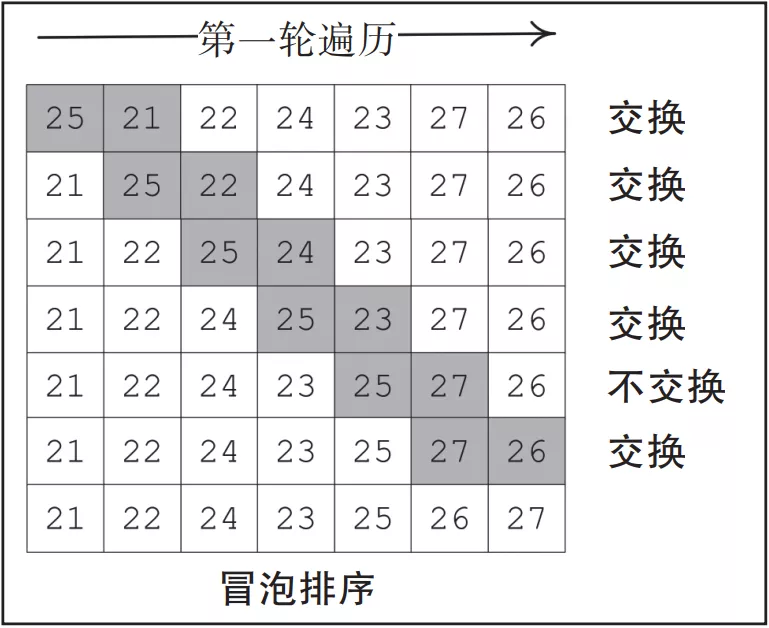▲图 3-2

``````#Pass 1 of Bubble Sort
lastElementIndex = len(list)-1
print(0,list)
for idx in range(lastElementIndex):
if list[idx]>list[idx+1]:
list[idx],list[idx+1]=list[idx+1],list[idx]
print(idx+1,list)``````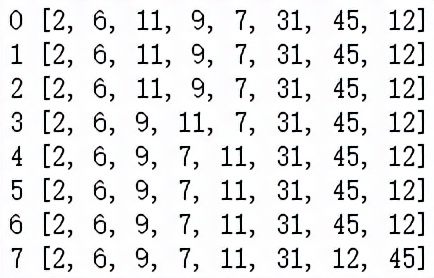▲图 3-3

``````def BubbleSort(list):
# Excahnge the elements to arrange in order
lastElementIndex = len(list)-1
for passNo in range(lastElementIndex,0,-1):
for idx in range(passNo):
if list[idx]>list[idx+1]:
list[idx],list[idx+1]=list[idx+1],list[idx]
return list``````

• 冒泡排序的性能

• 外层循环：外层循环称为遍历。例如，第一轮遍历就是外层循环的第一次迭代。
• 内层循环：在每次内层循环的迭代过程中，对列表中剩余的未排序元素进行排序，直到最高值冒泡到右侧为止。第一轮遍历将进行N–1次比较，第二轮遍历将进行N–2次比较，而每轮后续遍历将减少一次比较操作。

## 03 插入排序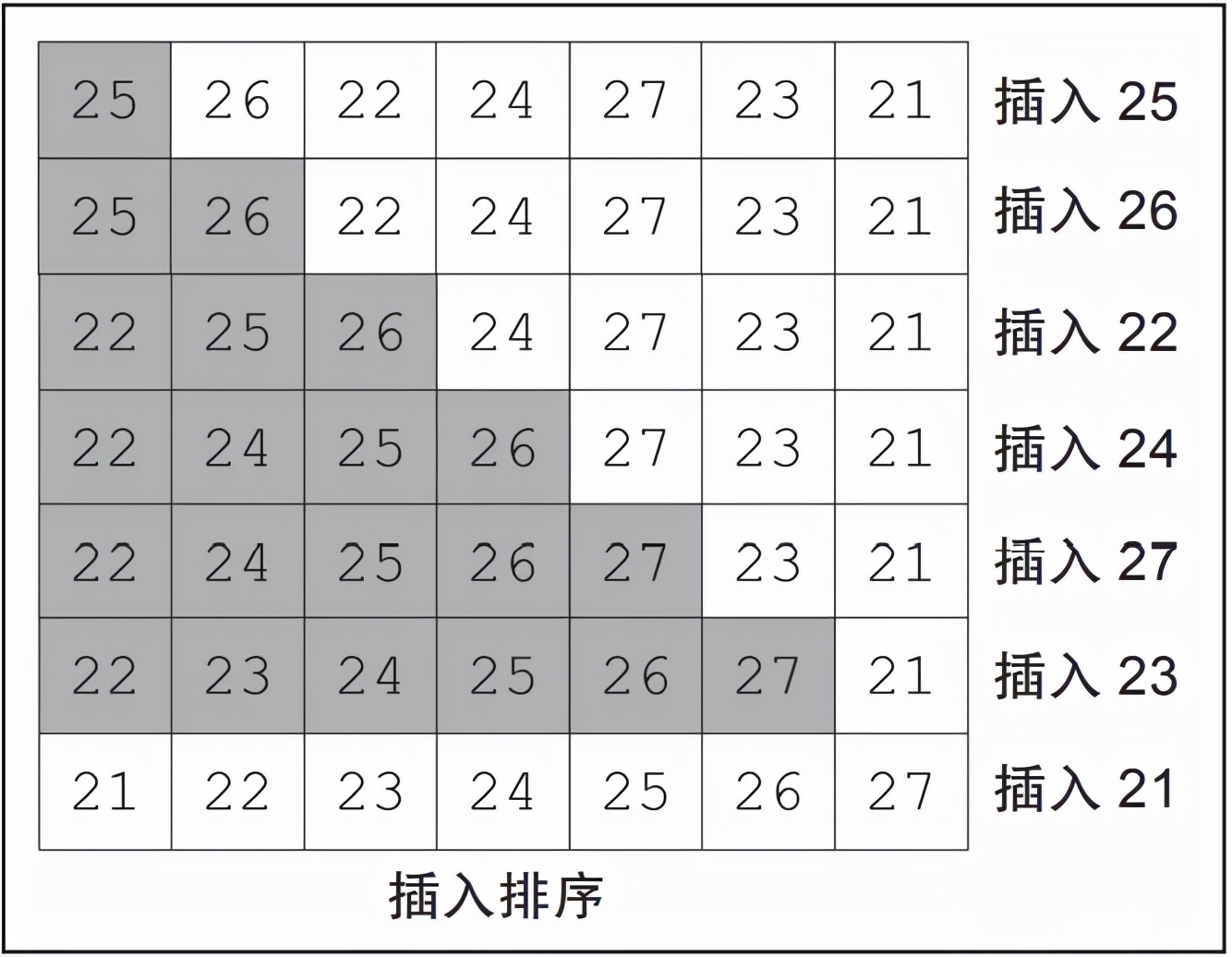▲图 3-5

``````def InsertionSort(list):
for i in range(1, len(list)):
j = i-1
element_next = list[i]
while (list[j] > element_next) and (j >= 0):
list[j+1] = list[j]
j=j-1
list[j+1] = element_next
return list``````

``````list = [25,26,22,24,27,23,21]

InsertionSort(list)
print(list)``````

``[21,22,23,24,25,26,27]``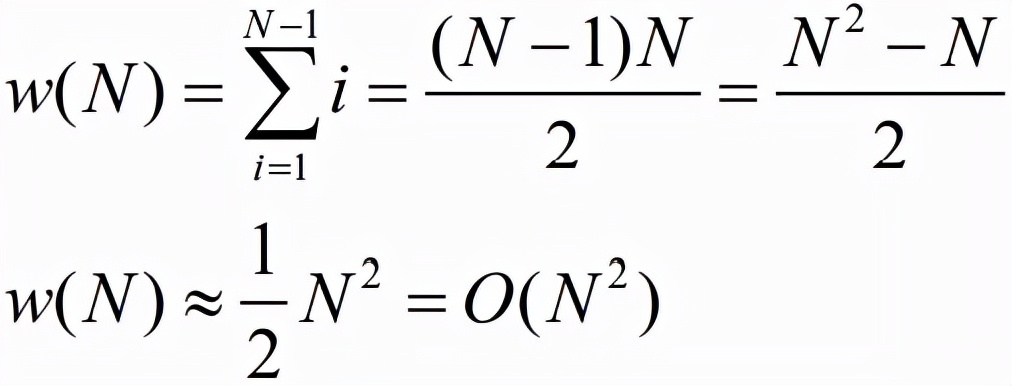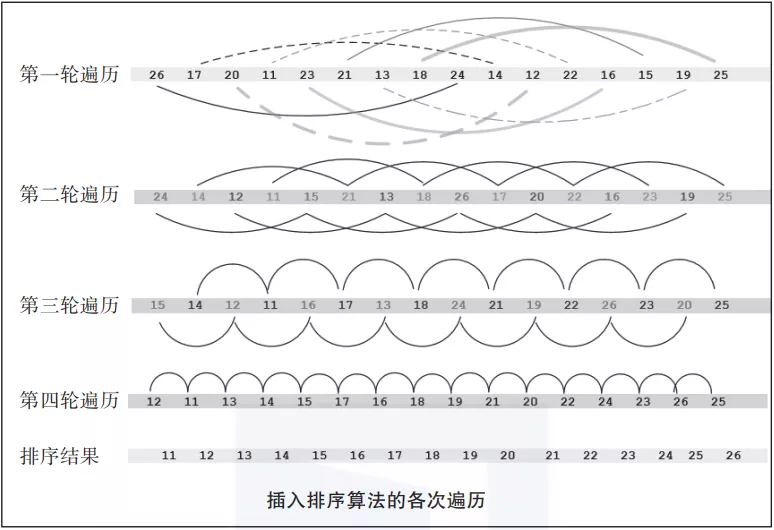▲图 3-7

## 04 归并排序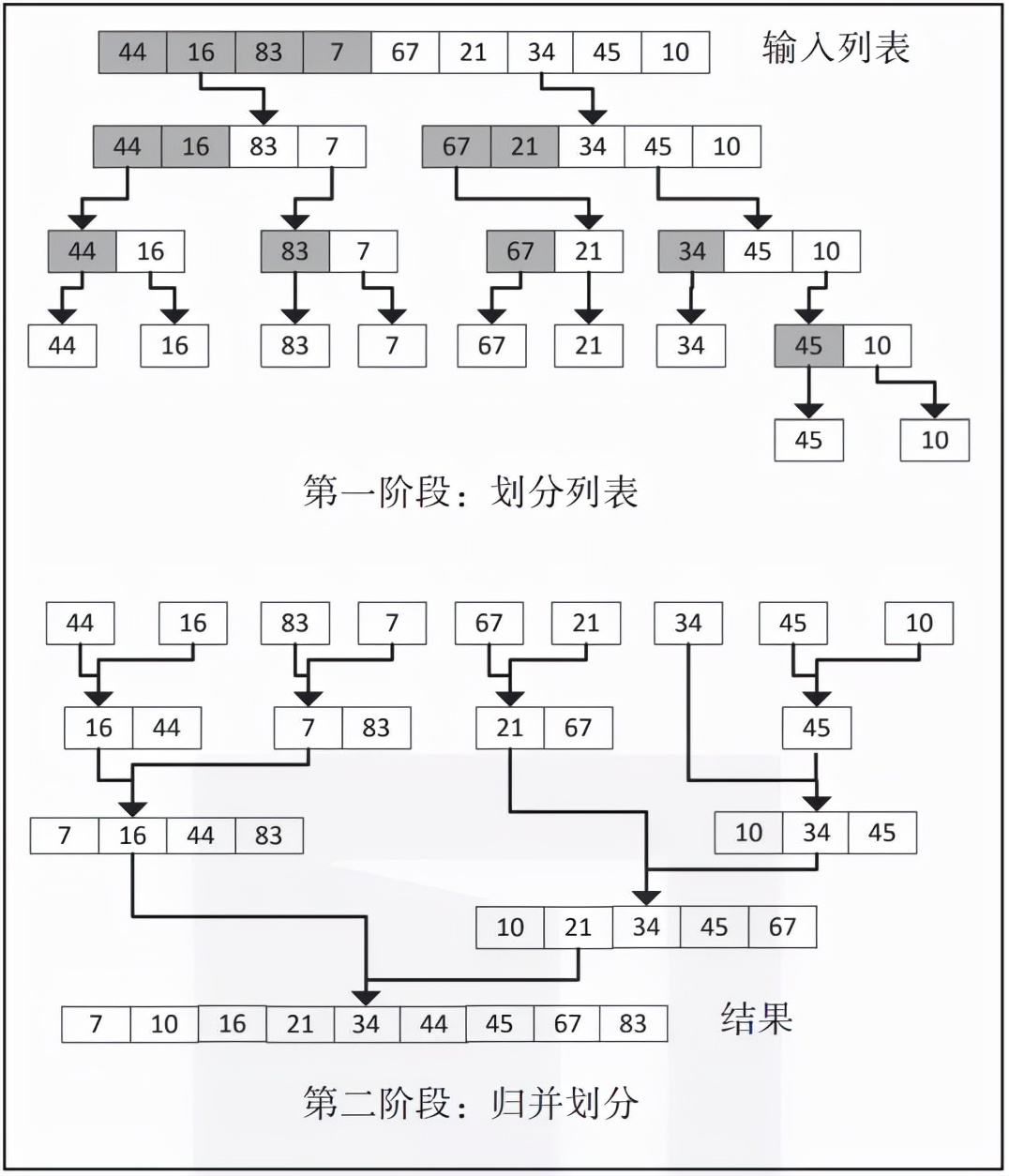▲图 3-8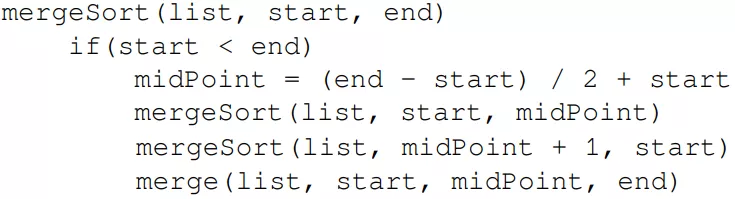1. 它将列表划分为两个长度相等的部分。
2. 它使用递归来进行划分，直到每个列表的长度为1。
3. 它将有序的部分归并成一个有序的列表并返回。

``````def MergeSort(list):
if len(list)>1:
mid = len(list)//2 #splits list in half
left = list[:mid]
right = list[mid:]

MergeSort(left) #repeats until length of each list is 1
MergeSort(right)

a = 0
b = 0
c = 0
while a < len(left) and b < len(right):
if left[a] < right[b]:
list[c]=left[a]
a = a + 1
else:
list[c]=right[b]
b = b + 1
c = c + 1
while a < len(left):
list[c]=left[a]
a = a + 1
c = c + 1

while b < len(right):
list[c]=right[b]
b = b + 1
c = c + 1
return list``````

``````list = [44,16,83,7,67,21,34,45,10]
MergeSort(list)
print(list)

[7, 10, 16, 21, 34, 44, 45, 67, 83]``````

## 05 希尔排序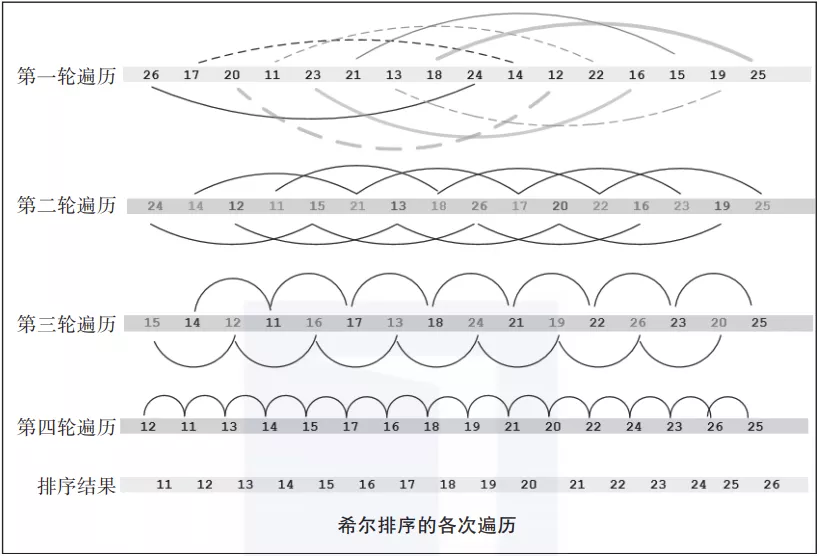▲图 3-11

``````def ShellSort(list):
distance = len(list) // 2
while distance > 0:
for i in range(distance, len(list)):
temp = list[i]
j = i
# Sort the sub list for this distance
while j >= distance and list[j - distance] > temp:
list[j] = list[j - distance]
j = j-distance
list[j] = temp
# Reduce the distance for the next element
distance = distance//2
return list``````

``````list = [26,17,20,11,23,21,13,18,24,14,12,22,16,15,19,25]
ShellSort(list)
print(list)

[11,12,13,14,15,16,17,18,19,20,21,22,23,24,25,26]``````

• 希尔排序的性能

## 06 选择排序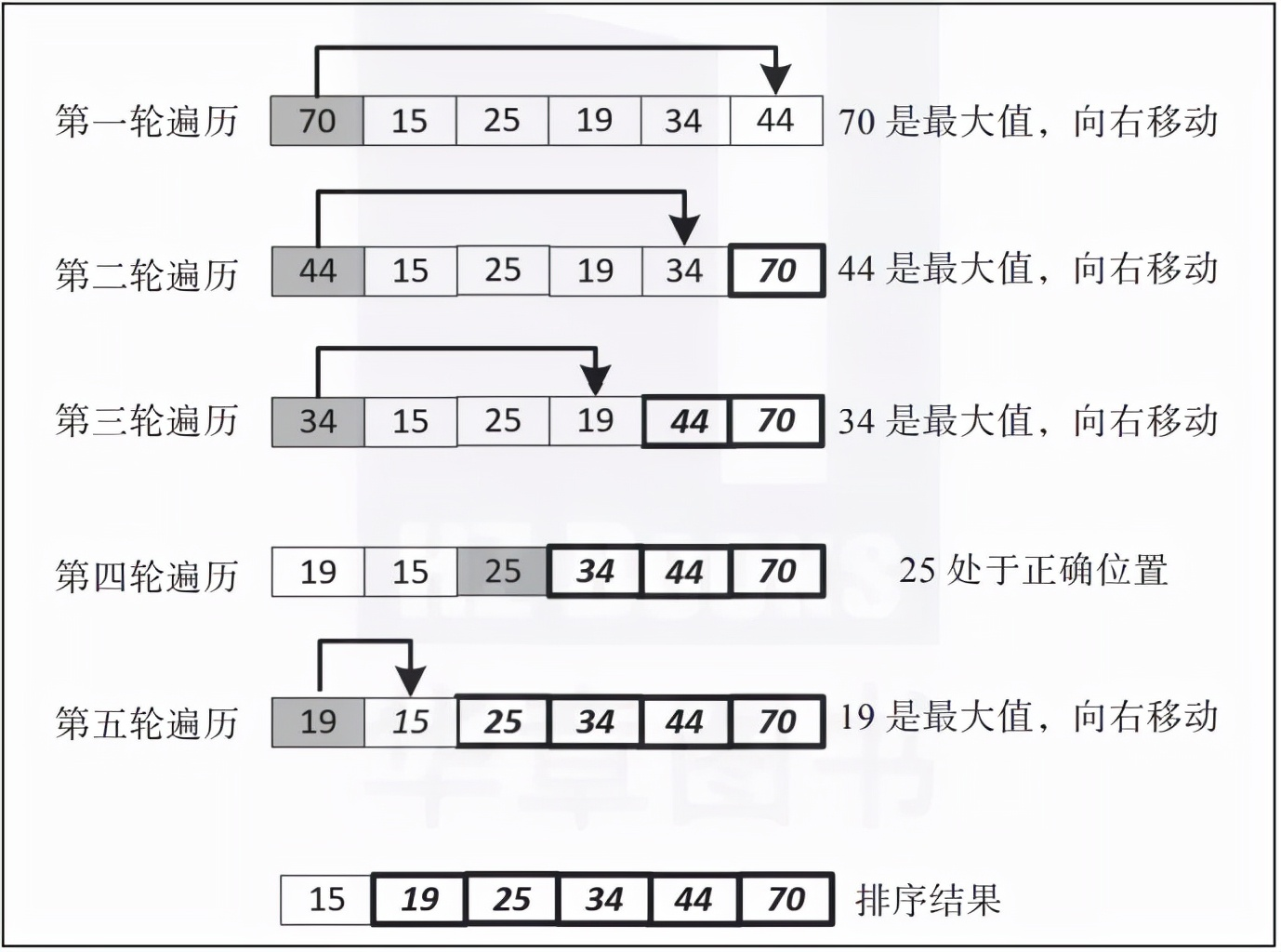▲图 3-13

``````def SelectionSort(list):
for fill_slot in range(len(list) - 1, 0, -1):
max_index = 0
for location in range(1, fill_slot + 1):
if list[location] > list[max_index]:
max_index = location
list[fill_slot],list[max_index] = list[max_index],list[fill_slot]
return list``````

``````list = [70,15,25,19,34,44]
SelectionSort(list)
print(list)

[15,19,25,34,44,70]``````

• 选择排序的性能

## 小结：选择一种排序算法热点精选